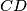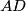### IMO Shortlist 1984 problem 14

Kvaliteta:
Avg: 0,0
Težina:
Avg: 0,0
Let$ABCD$ be a convex quadrilateral with the line$CD$ being tangent to the circle on diameter$AB$. Prove that the line$AB$ is tangent to the circle on diameter$CD$ if and only if the lines$BC$ and$AD$ are parallel.
Izvor: Međunarodna matematička olimpijada, shortlist 1984